International
Tables for
Crystallography
Volume A
Space-group symmetry
Edited by M. I. Aroyo

International Tables for Crystallography (2016). Vol. A, ch. 1.6, pp. 109-110

## Section 1.6.2.2. Structure-factor statistics and crystal symmetry

U. Shmuelia and H. D. Flackb

#### 1.6.2.2. Structure-factor statistics and crystal symmetry

| top | pdf |

Most structure-solving software packages contain a section dedicated to several probabilistic methods based on the Wilson (1949) paper on the probability distribution of structure-factor magnitudes. These statistics sometimes correctly indicate whether the intensity data set was collected from a centrosymmetric or noncentrosymmetric crystal. However, not infrequently these indications are erroneous. The reasons for this may be many, but outstandingly important are (i) the presence of a few very heavy atoms amongst a host of lighter ones, and (ii) a very small number of nearly equal atoms. Omission of weak reflections from the data set also contributes to failures of Wilson (1949) statistics. These erroneous indications are also rather strongly space-group dependent.

The well known probability density functions (hereafter p.d.f.'s) of the magnitude of the normalized structure factor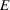, also known as ideal p.d.f.'s, are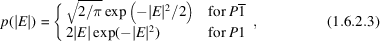where it is assumed that all the atoms are of the same chemical element. Let us see their graphical representations.

It is seen from Fig. 1.6.2.1that the two p.d.f.'s are significantly different, but usually they are not presented as such by the software. What is usually shown are the cumulative distributions of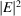, the moments: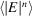for n = 1, 2, 3, 4, 5, 6, and the averages of low powers of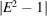for ideal centric and acentric distributions, based on equation (1.6.2.3). Table 1.6.2.2shows the numerical values of several low-order moments of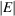and that of the lowest power of. The higher the value of n the greater is the difference between their values for centric and acentric cases. However, it is most important to remember that the influence of measurement uncertainties also increases with n and therefore the higher the moment the less reliable it tends to be.

 Table 1.6.2.2| top | pdf | The numerical values of several low-order moments of, based on equation (1.6.2.3)MomentP1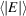0.798 0.886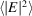1.000 1.000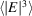1.596 1.329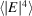3.000 2.000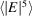6.383 3.323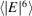15.000 6.0000.968 0.736Figure 1.6.2.1 | top | pdf |Ideal p.d.f.'s for the equal-atom case. The dashed line is the centric, and the solid line the acentric ideal p.d.f.

There are several ideal indicators of the status of centrosymmetry of a crystal structure. The most frequently used are: (i) the N(z) test (Howells et al., 1950), a cumulative distribution of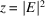, based on equation (1.6.2.3), and (ii) the low-order moments of, also based on equation (1.6.2.3). Equation (1.6.2.3), however, is very seldom used as an indicator of the status of centrosymmetry of a crystal stucture.

Let us now briefly consider p.d.f.'s that are valid for any atomic composition as well as any space-group symmetry, and exemplify their performance by comparing a histogram derived from observed intensities from astructure with theoretical p.d.f.'s for the space groups P1 and. The p.d.f.'s considered presume that all the atoms are in general positions and that the reflections considered are general (see, e.g., Section 1.6.3). A general treatment of the problem is given in the literature and summarized in the book Introduction to Crystallographic Statistics (Shmueli & Weiss, 1995).

The basics of the exact p.d.f.'s are conveniently illustrated in the following. The normalized structure factor for the space group, assuming that all the atoms occupy general positions and resonant scattering is neglected, is given by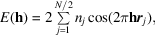where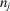is the normalized scattering factor. The maximum possible value ofis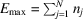and the minimum possible value ofis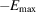. Therefore,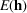must be confined to the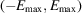range. The probability of finding E outside this range is of course zero. Such a probability density function can be expanded in a Fourier series within this range (cf. Shmueli et al., 1984). This is the basis of the derivation, the details of which are well documented (e.g. Shmueli et al., 1984; Shmueli & Weiss, 1995; Shmueli, 2007). Exact p.d.f.'s for any centrosymmetric space group have the form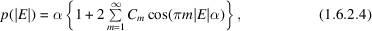where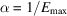, and exact p.d.f.'s for any noncentrosymmetric space group can be computed as the double Fourier series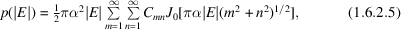where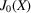is a Bessel function of the first kind and of order zero. Expressions for the coefficients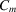and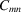are given by Rabinovich et al. (1991) and by Shmueli & Wilson (2008) for all the space groups up to and including.

The following example deals with a very high sensitivity to atomic heterogeneity. Consider the crystal structure of [(Z)-ethyl N-iso­propyl­thio­carbamato-κS]­(tricyclo­hexyl­phos­phine-κP)­gold(I), published aswith Z = 2, the content of its asymmetric unit being AuSPONC24H45 (Tadbuppa & Tiekink, 2010). Let us construct a histogram from thedata computed from all the observed reflections with non-negative reduced intensities and compare the histogram with the p.d.f.'s for the space groups P1 and, computed from equations (1.6.2.5)and (1.6.2.4), respectively. The histogram and the p.d.f.'s were put on the same scale. The result is shown in Fig. 1.6.2.2.Figure 1.6.2.2 | top | pdf |Exact p.d.f.'s. for a crystal of [(Z)-ethyl N-isopropylthiocarbamato-κS]­(tricyclohexylphosphine-κP)gold(I) in the triclinic system. Solid curve:, computed from (1.6.2.4); dashed curve: P1, computed from (1.6.2.5); histogram based on the data computed from all the reflections with non-negative reduced intensities. The height of each bin corresponds to the number of reflections (NREF) in its range ofvalues. The p.d.f.'s are scaled up to the histogram.

A visual comparison strongly indicates that the space-group assignment aswas correct, since the recalculated histogram agrees rather well with the p.d.f. (1.6.2.4)and much less with (1.6.2.5). The ideal Wilson-type statistics incorrectly indicated that this crystal is noncentrosymmetric. It is seen that the ideal p.d.f. breaks down in the presence of strong atomic heterogeneity (gold among many lighter atoms) in the space group. Other space groups behave differently, as shown in the literature (e.g. Rabinovich et al., 1991; Shmueli & Weiss, 1995).

Additional examples of applications of structure-factor statistics and some relevant computing considerations and software can be found in Shmueli (2012) and Shmueli (2013).

### References

Howells, E. R., Phillips, D. C. & Rogers, D. (1950). The probability distribution of X-ray intensities. II. Experimental investigation and the X-ray detection of centres of symmetry. Acta Cryst. 3, 210–214.
Rabinovich, S., Shmueli, U., Stein, Z., Shashua, R. & Weiss, G. H. (1991). Exact random-walk models in crystallographic statistics. VI. P.d.f.'s of E for all plane groups and most space groups. Acta Cryst. A47, 328–335.
Shmueli, U. (2007). Theories and Techniques of Crystal Structure Determination. Oxford University Press.
Shmueli, U. (2012). Structure-factor statistics and crystal symmetry. J. Appl. Cryst. 45, 389–392.
Shmueli, U. (2013). INSTAT: a program for computing non-ideal probability density functions of |E|. J. Appl. Cryst. 46, 1521–1522.
Shmueli, U. & Weiss, G. H. (1995). Introduction to Crystallographic Statistics. Oxford University Press.
Shmueli, U., Weiss, G. H., Kiefer, J. E. & Wilson, A. J. C. (1984). Exact random-walk models in crystallographic statistics. I. Space groupsand P1. Acta Cryst. A40, 651–660.
Shmueli, U. & Wilson, A. J. C. (2008). Statistical properties of the weighted reciprocal lattice. In International Tables for Crystallography, Volume B, Reciprocal Space, edited by U. Shmueli, ch. 2.1. Dordrecht: Springer.
Tadbuppa, P. P. & Tiekink, E. R. T. (2010). [(Z)-Ethyl N-isopropylthiocarbamato-κS](tricyclohexylphosphine-κP)gold(I). Acta Cryst. E66, m615.
Wilson, A. J. C. (1949). The probability distribution of X-ray intensities. Acta Cryst. 2, 318–321.